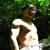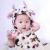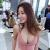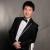## 百度可以ping通，但是打不开！请问怎么解决？谢谢

xzg 发布于 2012/07/18 12:07

为什么80%的码农都做不了架构师？->>>ping www.baidu.com -t

Pinging www.a.shifen.com [119.75.218.77] with 32 bytes of data

Reply from 119.75.218.77: bytes=32 time=44ms TTL=56
Reply from 119.75.218.77: bytes=32 time=44ms TTL=56
Reply from 119.75.218.77: bytes=32 time=45ms TTL=56
Reply from 119.75.218.77: bytes=32 time=44ms TTL=56
Reply from 119.75.218.77: bytes=32 time=44ms TTL=56
Reply from 119.75.218.77: bytes=32 time=45ms TTL=56
Reply from 119.75.218.77: bytes=32 time=44ms TTL=56
Reply from 119.75.218.77: bytes=32 time=44ms TTL=56
Reply from 119.75.218.77: bytes=32 time=44ms TTL=56
Reply from 119.75.218.77: bytes=32 time=45ms TTL=56
Reply from 119.75.218.77: bytes=32 time=44ms TTL=56
Reply from 119.75.218.77: bytes=32 time=44ms TTL=56
Reply from 119.75.218.77: bytes=32 time=45ms TTL=56
Reply from 119.75.218.77: bytes=32 time=46ms TTL=56
Reply from 119.75.218.77: bytes=32 time=43ms TTL=56
Reply from 119.75.218.77: bytes=32 time=44ms TTL=56
Reply from 119.75.218.77: bytes=32 time=44ms TTL=56
Reply from 119.75.218.77: bytes=32 time=45ms TTL=56
Reply from 119.75.218.77: bytes=32 time=44ms TTL=56
Reply from 119.75.218.77: bytes=32 time=44ms TTL=56
Reply from 119.75.218.77: bytes=32 time=46ms TTL=56
Reply from 119.75.218.77: bytes=32 time=44ms TTL=56
Reply from 119.75.218.77: bytes=32 time=45ms TTL=56
Reply from 119.75.218.77: bytes=32 time=44ms TTL=56

Ping statistics for 119.75.218.77:
Packets: Sent = 24, Received = 24, Lost = 0 (0% loss),
Approximate round trip times in milli-seconds:
Minimum = 43ms, Maximum = 46ms, Average = 44ms

0000

0